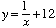Hi my name is Lori. I'm a student, in the 11th grade.I have a problem in quadratic functions. I don't know how to get the numbers into the problem and then how to do the problem. here is one problem the rest are sort of similar to it... y=2x squared. If I were to find out how to get the (x,y) answers then I could graph it but as I said I can't find the numbers to go into the problem.

Also in the equation g: x arrow to y = -x + 4..... how would you know weather it is or isn't a quadratic equation????

Thanks Much,

Lori

Hi Lori,

The expression y = 2 x2 describes the relationship between x and y. What is says is that if x is any number then y is twice the square of x. Thus if x is 3 then y is twice 32, that is y is 2*9 = 18 and if x is -1 then y is 2*(-1)2 = 2. Hence to "find the numbers to go into the problem" you get to choose x and then see what y results. Here are some more examples.

xy = 2 x2
318
-12
28
00

In your second question y = -x + 4 is not a quadratic equation since it doesn't have an x2 term. A quadratic equation is one with the form

y = a x2 + b x + c

where a, b, and c are numbers with a not being zero. Thus y = 3 x2 - 7, y = x2 + 5 x - 5 anf y = -9 x2 + 5 x are quadratics but y = 3 x + 9 andare not.

Cheers,
Penny
Go to Math Central